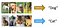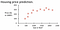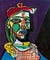# Expert Systems

As humans, we have a complex set of rules in our minds, based on which every single decision is made. These decisions are subjected to the influential factors which must be taken into consideration to ensure a positive outcome. Thus, if a machine has to be imbibed with this intelligent ability, we have two alternatives.

1. Provide the complex set of rules in form of code. (Not optimal)
2. Use Machine Learning. (Oh yeah!)

We ourselves, do not comprehend our train of thoughts, thus it is safe to assume the implausible prospect of making an Artificial Brain by coding decision rules into a program. Which is why, we switch to the alternative path of Machine Learning.

# Jars of Machine Learning

Jars? Well, that’s because everybody loves Jam and it is short for Jargon. These jars represent the 6 primary components required to successfully implement machine learning.

# Data

From a high level perspective, a machine learning algorithm can be viewed as a function that accepts ‘X’ and spits out ‘Y’.

‘X’ is an ordered tuple of numerical values. Since, machine learning has it’s roots in calculus and algebra, it only works with numbers. Every numerical value is the quantitative representation of an attribute/feature. The machine learning algorithms take these attributes into consideration to determine the correct outcome.

Usually, the data also includes the corresponding outcome that the algorithm is expected to produce, which is ‘Y’. This kind of data set is known as Labeled data.

# Task

Task is the objective that the machine learning algorithm seeks to accomplish. They can be broadly classified into Classification, Regression and Generation.Image from Edureka

Is it a Dog or a Cat? The task here is to identify if the image provided is that of a dog or a cat. Image classification is popularly performed using Convolution Neural Networks.Image from pybros.com

House Price Prediction. The task here is to predict the price of a house, given the features and specifications like location, area, number of rooms etc.Hmm.. Beautiful?

Creating Fake Picasso paintings. Yeah, that’s right! We can actually produce art mimicking Picasso’s style. Generative Adversarial Neural Networks are used for this purpose.

# Model

Models represent machine learning algorithms which can be used to successfully perform the chosen task. There are numerous models to choose from, and the choice is made using the bias-variance trade off. The bias represents the inability of the model to successfully mold to the data and the variance represents the variation in outcome when the model is applied to the unseen data. Both have detrimental affects on the task at hand.

Bias and variance are related to each other by inverse proportionality, known as Bias-Variance trade off. Thus, the optimal model we choose must lie at the sweet spot, where the bias and variance are low.

Examples of simple models in Regression with increasing complexity are

Y = AX + C {A Straight Line}

Y = AX² + BX + C {A Parabola}

Y = AX²³ + BX²² + … +Z {Wavy.. Very Wavy}

# Loss

Y = AX² + BX + C

The performance of a model is characterized by the parameters associated with it. For example, the coefficients A, B and constant C are the parameters to the above model and can take any numerical value. To determine the optimal model parameters, we must define a quantitative metric for the fitness of the model with the data. This calls for a Loss function.

The most common loss function is the Sum Squared Error (SSE), which is the squared difference between the Expected outcome and the Observed outcome.

# Learning

The optimal model parameters are determined using a learning algorithm. It is extremely taxing for a machine to compute the optimal parameters of a model using brute force, which is why several learning algorithms with roots in calculus have been developed to efficiently compute these parameters.

Some of these are Gradient Descent, Back Propagation (BP) in Feed Forward Neural Networks and Back Propagation Through Time (BPTT) in Recurrent Neural Networks.

# Evaluation

Usually, the data we employ for machine learning is incomplete and is a sample from a huge population. There is a possibility that the model we train molds into the data to an undesirable extent, i.e., it learns rules specific to the sample and not the population as a whole, this is called Over-fitting.

We do not wish for our model to be specific to the training data. Generality is essential if the model is to perform optimally on the remaining data from the population and thus rises the requirement of Evaluating models.

Some popular methods for evaluating models are Cross Validation and Hold Out method. In case of classification, we have additional evaluation criteria called Precision and Recall.

# Post Word

Thanks for reading! Writing this blog has helped me consolidate my ideas and understand the unique model of 6 Jars explained in the Deep Learning Course of PadhAI by One Fourth Labs and I hope it has been an enjoyable read which highlights the gist of machine learning. What do you think about these 6 Jars? Let me know in the comments :)

Just a Geek